# SSC CGL 2013 Tier 1 21 April Morning Shift

Instructions

For the following questions answer them individually

Question 191

In the given figure, Circle represents persons having Car, triangle represents persons having Motor Cycle, square represents persons having Autorickshaws, rectangle represents persons having Cycle. Find the region where persons having Car, Motor Cycle, Cycle but not Autorickshaw.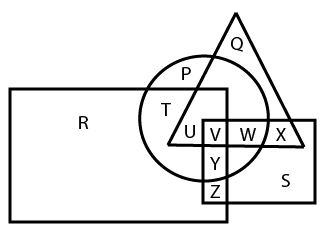Question 192

Identify the response figure in which the figures given are found.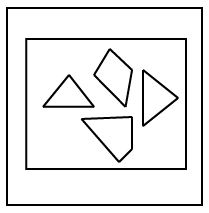Instructions

In the following questions, study the following diagram carefully and answer the questions based on it.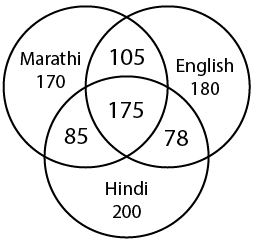Question 193

The diagram shows the survey on a sample of 1000 persons with reference to their knowledge of English, Hindi and Marathi. How many know all the languages ?

Question 194

The diagram shows the survey on a sample of 1000 persons with reference to their knowledge of English, Hindi and Marathi. 105 people know…….languages.

Instructions

In the following questions, which answer figure will complete the pattern in the question figure ?

Question 195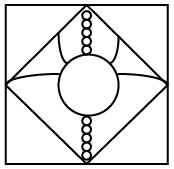Question 196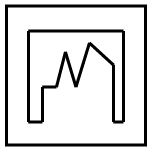Instructions

For the following questions answer them individually

Question 197

A piece of paper is folded and punched as shown below in the question figures.From the given answer figures, indicate how it will appear when opened.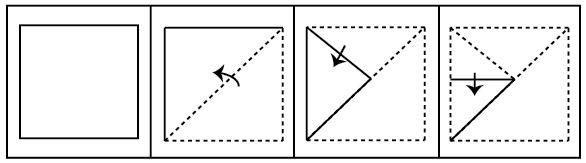Question 198

A word is represented by only one set of numbers as given in any one of the alternatives. The sets of numbers given in the alternatives are represented by two classes of alphabets as in two matrices given below. The columns and rows of Matrix I are numbered from 0 to 4 and that of Matrix II are numbered from 5 to 9 A letter from these matrices can be represented first by its row and next by its column, e.g., ‘N’ can be represented by 02, 24 etc. and ‘Q’ can be represented by 56, 78 etc. Similarly, you have to identify the set for the word ‘SPORTS’.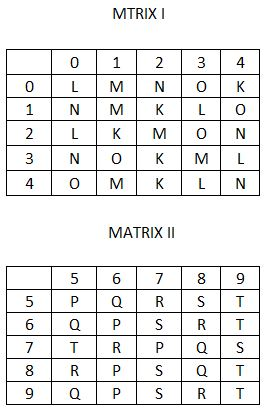Question 199

If a mirror is placed on the line XY then which of the answer figures is the right image of the given figure ?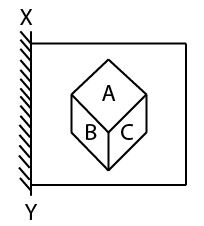Question 200

From the given answer figures, select the one in which the question figure is hidden/ embedded.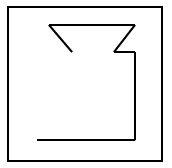OR